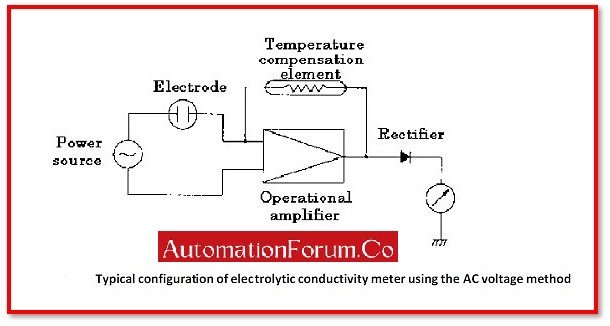# What is Conductivity Meter?# What is Conductivity Meter?

Measurements of electrical current or conductance in a solution are measured using a conductivity meter. When measuring the general condition of a natural water body, conductivity is important. In water treatment plants, it is also a way to track changes to the way wastewater is processed.

## What is the SI unit of Conductivity?

The SI unit of Conductivity is Siemens per meter (S/m).

## What is the Symbol of Conductivity?

The symbol of Electrical Conductivity:

## What is the basic principle of a Conductivity Meter?

The capacity of a material to carry an electric current is known as conductivity. Instruments use a principle to test conductivity: two plates are placed in the sample; a potential is applied across them and typically a sine wave voltage and the amount of current that flows through the mixture is measured.

Where,

k- Conductivity of Solution

K- Constant determined by the electrode

R- Resistance of Solution

## What is the nomenclature of the Conductivity Meter?

The electrical conductivity of a solution is measured using an electrical conductivity meter. It is frequently used in hydroponic, aquaculture, aquaponic, and freshwater systems to monitor the concentration of nutrients, salts, or pollutants in the water. It has numerous uses in research and engineering.

## What is the use of a Conductivity Meter?

The ability of a material (such as solutions, metals, or gases) to conduct electricity is known as conductivity. Although all materials can conduct electric currents, the strength of this ability might vary.

## The Operation of a Conductivity Meter:

The meter has a probe that can be used for field or on-site measurements; this probe is often portable. The meter applies voltage between two electrodes inside the probe once it has been inserted into the liquid to be measured. The solution’s electrical resistance results in a drop in voltage that the meter detects. This reading is converted by the meter into milli-, micro-, or micro-Siemens per centimetre. This number represents all dissolved solids. The quantity of particles that can flow through a glass-fibre filter is known as total dissolved solids.

## Measuring Method of Conductivity Method:

Measuring Method includes 2 types:

1. Conductivity Meter using Alternating Bipolar Method and
2. Conductivity Meter using Electromagnetic Induction Method

### Conductivity Meter using Alternating Bipolar Method:

To determine its electrolytic conductivity, a two-pole electrode is immersed in a solution. The two poles receive an input of AC voltage. The resistance (R) and electrolytic conductivity of the solution are both measured using the electric current passing between the poles. Since the electrolytic conductivity changes with temperature, a temperature adjustment device is used to adapt the measured electrolytic conductivity to that at 25°C.

### Conductivity Meter using Electromagnetic Induction Method:

Transformers T1 and T2 are used to create two electrodes, which are immersed in a solution. When an alternating current (AC) is applied to the primary coil C1, an induced current (I) proportional to the electrolytic conductivity of the solution flows through C2. On the other hand, when the transformer T2 uses coil C2 as its main coil, voltage E is produced in the secondary coil C3 in proportion to the induced current I. The electrolytic conductivity is measured using this voltage.

1. Conductivity meter are portable, using them in a lab or on a workplace is simple.

2. Conductivity meter processes its data using a microprocessor, its detection accuracy is also quite good.

3. Results are easily viewed on an LCD screen, allowing the inspector to do so without making efforts.

4. Conductivity meter include accessories that are simple to link with the majority of automation systems, automation is feasible.

5. Because the test sample is kept intact before, during, and after the test, it is particularly cost-effective as a non-destructive testing tool.

1. Conductivity meters unable to differentiate between different ion types.

2. Conductivity meters are temperature-dependent; conductance rises by around 2% every degree ℃.

3. Conductivity meters does not accurately measure the ions present in a solution.

## Applications of Conductivity Meter:

1. The measurement of conductivity is a flexible process control tool.

2. To assess the homogeneity of the alloy components, an EC meter is utilised for alloys.

3. An electrical conductivity meter can also be used to determine a material’s current resistance.

4. EC meter can be used to test non-ferrous metals’ conductivity, such as copper and aluminium.

5. An electrical conductivity meter is used to track the heat treatment process during the hardening and heat working of metals.

Scroll to Top